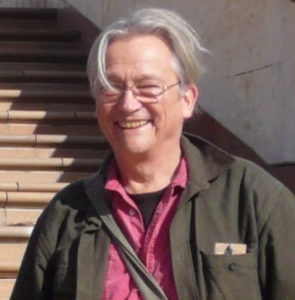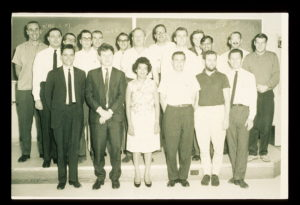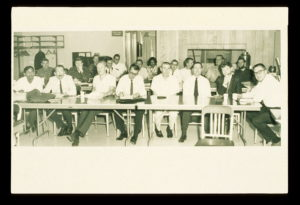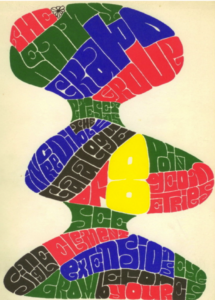# Henry Crapo: A Brief Reminiscence

A guest post by James Oxley.Henry in Wellington in 2007

Henry Crapo died on September 3, 2019 at the age of 86. He contributed much to matroid theory, making foundational contributions to the subject. Among his notable achievements were the first text in matroid theory, written jointly with Gian-Carlo Rota; the introduction of the Tutte polynomial and the beta invariant for matroids; the analysis of how to treat single-element extensions of matroids; the identification of the critical problem for matroids; the definition of an attractive non-commutative way to combine two matroids; and a catalogue of all matroids on at most eight elements. He was a very friendly and generous man who, in addition to his many influential publications, hosted intimate and stimulating conferences at his home in the south of France. He will be greatly missed.

This short remembrance will highlight some of Henry’s most important contributions to matroid theory. In 1964, Henry completed his Ph.D. dissertation at MIT. According to the online Mathematics Genealogy Project, Henry had two advisors: Gian-Carlo Rota and Kenneth Myron Hoffmann, although more will be said about this below. Rota’s name will be well known to matroid theorists (or “combinatorial geometers” as he would have preferred), but Hoffman is unlikely to be as he worked in functional analysis.

Henry was one of the attendees at the first conference in matroid theory, which was organized by Jack Edmonds and held at the National Bureau of Standards in Washington, D.C. in 1964. Bill Tutte was another notable attendee at that conference where he delivered his Lectures on Matroids. Of that year, Tutte wrote, To me that was the year of the Coming of the Matroids. Then and there the theory of matroids was proclaimed to the mathematical world. And outside the halls of lecture there arose the repeated cry: “What the hell is a matroid?”.’

Henry’s first paper , which was on single-element extensions of matroids, appeared in the proceedings of that conference, which were published in 1965 in the Proceedings of the National Bureau of Standards. In that paper, Henry noted that, when extending a matroid $M$ by an element $e$ to produce a new matroid $N$, one needs only to consider the flats $F$ of $M$ such that $F\cup e$ is a flat of $N$ with $r(F \cup e) = r(F)$. The set ${\mathcal M}$ of such flats $F$ is called a modular cut. Thus, for example, if ${\mathcal M}$ is empty, then $e$ is added as a coloop, whereas if ${\mathcal M}$ consists of all of the flats of $M$, then $e$ is added as a loop. The extension $N$ is uniquely determined by the modular cut ${\mathcal M}$. Thus a study of the single-element extensions of $M$ is a study of the modular cuts of $M$. Henry proved that a subset ${\mathcal F}$ of the flats of $M$ is a modular cut if and only if every flat containing a member of ${\mathcal F}$ is also in ${\mathcal F}$, and, whenever $X$ and $Y$ are in ${\mathcal F}$ and $r(X) + r(Y) = r(X \cup Y) + r(X \cap Y)$, the flat $X \cap Y$ is also in ${\mathcal F}$.

The pictures in Figures 1 and 2 are from that first matroid conference and were kindly supplied to me by Bill Pulleyblank. In the first one, Henry is in the front row on the extreme left. Slightly to his left in the row behind him is Gian-Carlo Rota. Bill Tutte is in the same row as Rota but two along from him. Jack Edmonds is in the back row, to Tutte’s immediate right. That photo also includes Tutte’s students, Neil Robertson and Ron Mullin, as well as Ray Fulkerson and Dijen Ray-Chaudhuri. Since the reader may enjoy trying to identify those people, their locations will not be revealed until the end of this note.Figure 1: Participants in the first matroid theory conference.Figure 2: The matroid theory conference participants seated.

Henry’s second and third papers [4, 5] appeared in Volumes 1 and 2 of the newly founded Journal of Combinatorial Theory. His third paper A higher invariant for matroids introduced what Brylawski [2, p.252] called “Crapo’s Betsy invariant”. More formally, for a matroid $M$, the beta invariant is defined by $$\beta (M) = (-1)^{r(M)} \sum_{A \subseteq E(M)} (-1)^{|A|} r(A).$$

Henry proved that, in a matroid $M$, for any element $e$ other than a loop or a coloop, $$\beta (M) = \beta(M\backslash e) + \beta(M/e).$$ Since $\beta(U_{0,1}) = 0$ and $\beta(U_{1,1}) = 1$, a straightforward induction argument gives that $\beta (M) \ge 0$ for all matroids $M$. Henry proved that, when $M$ has at least two elements, $M$ is connected if and only if $\beta(M)$ is positive. He also showed, again when $M$ has at least two elements, that $\beta(M) = \beta(M^*)$. Then, using Tutte’s excluded-minor characterization of regular matroids, Henry proved the following result.

Theorem. A matroid $M$ is regular if and only if $\beta(N_4) \le 1$ for all $4$-element minors $N_4$ of $M$, and $\beta(N_7) \le 2$ for all $7$-element minors $N_7$ of $M$.

Henry’s 1969 paper  The Tutte polynomial introduced the Tutte polynomial for matroids by building on Tutte’s 1954 paper  A contribution to the theory of chromatic polynomials, which introduced what we now call the Tutte polynomial for graphs. In a footnote in Henry’s paper, he observes that Sections 3 and 4 of his paper “constitute a rewriting, with new proofs, of the main theorems in the author’s doctoral dissertation On the Theory of Combinatorial Independence’, submitted to the Massachusetts Institute of Technology in June, 1964, under the supervision of Professor Gian-Carlo Rota.” Note that there is no mention here of Henry’s second advisor Kenneth Hoffman leading one to wonder what role he played.

Tutte himself studied the Tutte polynomial for representable matroids, which he called “nets”, in his 1948 Cambridge Ph.D. thesis . Since representability was not important in Tutte’s definition, he had effectively treated the Tutte polynomial for all matroids although he never published this work. The Tutte polynomial for matroids has been extensively studied by many authors in the half century since Henry’s paper formally introducing it. It can be defined as follows:
$$T(M;x,y) = \sum_{A \subseteq E} (x-1)^{r(E) – r(A)}(y-1)^{|A| – r(A)}.$$ Thus $T(U_{0,1};x,y) = y$ and $T(U_{1,1};x,y) = x$. This polynomial obeys the following two basic recursions: $$T(M;x,y) = T(M|\{e\};x,y) T(M\backslash e;x,y) ~~~\text{when e is a loop or a coloop,}$$ and $$T(M;x,y) = T(M\backslash e;x,y) + T(M/ e;x,y) ~~~\text{otherwise.}$$
It follows from these that we can write $$T(M;x,y) = \sum_{i,j\ge 0} t_{ij}x^iy^j$$ where $t_{ij} \ge 0$ for all $i$ and $j$. It turns out that the beta invariant shows up among these coefficients. Specifically, when $M$ has at least two elements, $$\beta(M) = t_{10} = t_{01}.$$

Beginning in 1964, Gian-Carlo Rota published a series of papers “On the foundations of combinatorial geometry.” The most widely cited paper in that series is Part I: Theory of Möbius functions . Part II, joint with Henry, is Combinatorial geometries and was published in 1970. That 25-page paper  was followed in the same year by a monograph whose full title is On the Foundations of Combinatorial Theory: Combinatorial Geometries. Preliminary Edition. This was the first text in matroid theory and, as such, was very influential. A year later, Tutte published An Introduction to the Theory of Matroids, which was effectively a reprinting of his 1965 Lectures on Matroids, but that book  did not attract nearly the same attention as Crapo and Rota’s book. When Crapo and Rota decided to update their book, it began as a joint project with Neil White [18, p.xv]. It turned into a series of three books [18, 19, 20], which were edited by Neil White and which contained chapters by a large number of different authors including two in the first volume by Henry. The approach to matroid theory taken by Crapo and Rota was a very geometric one and much of the focus was on the lattice of flats. Studying such geometric lattices is, of course, equivalent to studying simple matroids. With hindsight, the major drawback of this approach is that the geometric lattice of the dual of a matroid $M$ is not easily obtained from the geometric lattice of $M$. The fundamental role of duality and the link that it provides between deletion and contraction would seem to have been a major factor in the subsequent shift in focus in the study of matroids away from the study of geometric lattices. Crapo and Rota’s book has many attractive features. One in particular is the introduction of the critical problem for matroids. The goal of that problem was to provide a common framework within which one could view a number of problems in extremal combinatorics including what was then the Four Colour Problem, the Five-Flow Conjecture , and Tutte’s Tangential $2$-Block Conjecture . For a matroid $M$, the characteristic or (chromatic) polynomial is defined by $$p(M;\lambda) = \sum_{A \subseteq E} (-1)^{|A|}\lambda^{r(M) – r(A)}.$$ This is an evaluation of the Tutte polynomial: $$p(M;\lambda) = (-1)^{r(M)}T(M;1-\lambda,0).$$ If $G$ is a graph with $k(G)$ connected components and cycle matroid $M(G)$, the chromatic polynomial $P_G(\lambda)$ of the graph satisfies $$P_G(\lambda) = \lambda^{k(G)}p(M(G);\lambda).$$ Moreover, if $M$ is loopless and its underlying simple matroid is $M’$, then $p(M’;\lambda) = p(M;\lambda)$.

Now let $M$ be a rank-$r$ simple $GF(q)$-representable matroid and view $M$ as a restriction of the projective geometry $PG(r-1,q)$. When $q$ is at least five, the embedding of $M$ in $PG(r-1,q)$ need not be unique. The critical exponent (or critical number) $c(M;q)$ of $M$ is $r – k$ where $k$ is the rank of the largest projective subspace of $PG(r-1,q)$ that contains no element of $E(M)$. In particular, recalling that the affine geometry $AG(r-1,q)$ is obtained from $PG(r-1,q)$ by deleting the elements of a hyperplane of the latter, we see that $c(M;q) = 1$ if and only if $M$ is a restriction of $AG(r-1,q)$. It is natural to expect that $c(M;q)$ may depend on the embedding of $M$ in $PG(r-1,q)$. However, the following attractive result of Crapo and Rota  proves that it does not.

Theorem. Let $M$ be a rank-$r$ simple $GF(q)$-representable matroid. Then
$$c(M;q) = \min\{j \ge 1: p(M;q^j) > 0\}.$$
One consequence of this is that a simple graph $G$ is bipartite if and only if its critical exponent $c(M;2)$ is one. More generally, the chromatic number $\chi(G)$ of $G$ and the critical exponent of $M(G)$ obey the following: $$q^{c(M;q) – 1} < \chi(G) \le q^{c(M;q)}.$$ The study of the behaviour of the critical exponent of matroids has recently been enjoying a renaissance with it now being more commonly known as the critical number. Peter Nelson  has recently written a very approachable survey of some of this work.

In 1973, with John Blackburn and Denis Higgs, Henry published A Catalogue of Combinatorial Geometries . The preprint of that had an extraordinary cover, which is reprinted in Figure 3. The catalogue was assembled by doing sequences of single-element extensions. Thanks to Rudi Pendavingh and Stefan van Zwam, we have a wonderful matroid package in SageMath. This paper of Henry and his collaborators seems to have been the first to do extensive computation involving small matroids.Figure 3: Can you identify what this says? The answer appears below.

I met Henry when I was working with Tom Brylawski in North Carolina probably in 1980. By then, Henry’s research focus was on structural rigidity, a topic that occupied his mind for many years. Henry did return to work on matroids from time to time after that. I conclude this reminiscence with one such return, in 2005, when Henry and Bill Schmitt  introduced a beautiful and useful matroid construction. Let $M_1$ and $M_2$ be matroids on disjoint sets $E_1$ and $E_2$. One easy way to combine $M_1$ and $M_2$ is to take their direct sum, a matroid of rank $r(M_1) + r(M_2)$. Crapo and Schmitt defined a different matroid on $E_1 \cup E_2$ of rank $r(M_1) + r(M_2)$. The free product $M_1 \Box M_2$ of $M_1$ and $M_2$ has as its bases all subsets $B$ of $E_1 \cup E_2$ of cardinality $r(M_1) + r(M_2)$ such that $B\cap E_1$ is independent in $M_1$ while $B\cap E_2$ is spanning in $M_2$. This operation is non-commutative. Indeed, $M_1 \Box M_2 = M_2 \Box M_1$ if and only if both $M_1$ and $M_2$ have rank zero, or both $M_1^*$ and $M_2^*$ have rank zero. This operation has a number of attractive properties. For example, $$(M_1 \Box M_2)^* = M_2^* \Box M_1^*.$$ Moreover, given $|E_1|$, the matroid $M_1 \Box M_2$ uniquely determines $M_1$ and $M_2$ up to isomorphism.

Theorem. For matroids $M_1$, $M_2$, $N_1$, and $N_2$, if $M_1 \Box M_2 \cong N_1 \Box N_2$ and $|E(M_1)| = |E(N_1)|$, then $M_1 \cong N_1$ and $M_2 \cong N_2$.

In 1969, Dominic Welsh  made a natural and seemingly innocuous conjecture about the number $f(n)$ of non-isomorphic matroids on an $n$-element set. Thirty-five years later, the conjecture was settled essentially simultaneously by Manoel Lemos  and by Crapo and Schmitt  using quite different methods. Crapo and Schmitt’s proof of Dominic’s conjecture was derived by applying the last theorem.

Theorem. For all non-negative integers $m$ and $n$, $$f(m+n) \ge f(m)f(n).$$

Henry liked to host small meetings at his home in La Vacquerie in the south of France. These were very stimulating meetings. The mathematics was exciting and the food was superb, with Henry hiring a chef for the week. Henry had a wonderful cellar I am told, and he shared extensively from it during these meetings. In addition, he arranged for local cultural events in the evenings including music recitals and interactive puppet shows. The experience was unforgettable for all lucky enough to enjoy it. At the only such meeting I attended, Henry gave up his own bedroom for my use. He was a most generous man. Those of us fortunate enough to have known him will miss him greatly; and all of us have his extensive mathematical legacy to continue to enrich our lives.

In the photograph in Figure 1, Ray Fulkerson is in the back row on the extreme right; next to him is Neil Robertson; on the row in front of that, Ron Mullin is on the exteme right and Dijen Ray-Chaudhuri is next to him. In the photograph in Figure 2, Jack Edmonds is at the right-hand end of the table; two along from him is Gian-Carlo Rota; three along from him are, in order, Bill Tutte, Ray Fulkerson, and Dijen Ray-Chaudhuri. In the second row, starting at the left-hand end, the first three people are Ron Mullin, Neil Robertson, and Henry Crapo. In Figure 3, the title of the preprint is The Henry Crapo Group Presents The Incredible Catalogue of 8 Point Geometries. See Single Element Extensions Grow Before Your Eyes.

References

 Blackburn, John E.; Crapo, Henry H.; Higgs, Denis A. A catalogue of combinatorial geometries. Math. Comp. 27 (1973), 155–166.

 Brylawski, Thomas H. A decomposition for combinatorial geometries. Trans. Amer. Math. Soc. 171 (1972), 235–282.

 Crapo, Henry H. Single-element extensions of matroids. J. Res. Nat. Bur. Standards Sect. B 69B (1965), 55–65.

 Crapo, Henry H. The Möbius function of a lattice. J. Combinatorial Theory 1 (1966), 126–131.

 Crapo, Henry H. A higher invariant for matroids. J. Combinatorial Theory 2 (1967), 406–417.

 Crapo, Henry H. The Tutte polynomial. Aequationes Math. 3 (1969), 211–229.

 Crapo, Henry H.; Rota, Gian-Carlo On the foundations of combinatorial theory. II. Combinatorial geometries. Studies in Appl. Math. 49 (1970), 109–133.

 Crapo, Henry H.; Rota, Gian-Carlo. On the Foundations of Combinatorial Theory: Combinatorial Geometries. Preliminary Edition. M.I.T. Press, Cambridge, Mass.-London, 1970.

 Crapo, Henry; Schmitt, William. The free product of matroids. European J. Combin. 26 (2005), 1060–1065.

 Lemos, Manoel. On the number of non-isomorphic matroids. Adv. in Appl. Math. 33 (2004), 733–746.

 Nelson, Peter Colouring without colours: graphs and matroids. Lond. Math. Soc. Newsl. No. 482 (2019), 25–29.

 Rota, Gian-Carlo. On the foundations of combinatorial theory. I. Theory of Möbius functions. Z. Wahrscheinlichkeitstheorie und Verw. Gebiete 2 (1964), 340–368.

 Tutte, W. T. An algebraic theory of graph colorings. Ph.D. thesis. University of Cambridge, 1948.

 Tutte, W. T. A contribution to the theory of chromatic polynomials. Canadian J. Math. 6 (1954), 80–91.

 Tutte, W. T. Lectures on matroids. J. Res. Nat. Bur. Standards Sect. B 69B (1965), 1–47.

 Tutte, W. T. Introduction to the Theory of Matroids. American Elsevier, New York, 1971.

 Welsh, D. J. A. Combinatorial problems in matroid theory. Combinatorial Mathematics and its Applications (Proc. Conf., Oxford, 1969) pp. 291–306, Academic Press, London, 1971.

 White, Neil (editor). Theory of Matroids. Cambridge University Press. Cambridge. 1986.

 White, Neil (editor). Combinatorial Geometries. Cambridge University Press. Cambridge. 1987.

 White, Neil (editor). Matroid Applications. Cambridge University Press. Cambridge. 1992.

## 6 thoughts on “Henry Crapo: A Brief Reminiscence”

1.Federico Ardila on said:

Thank you for a great reminiscence.
Do you (or anyone) know who is the woman in the middle of the photo?

2.James Oxley on said:

Following Federico’s question, I tried to identify as many as I could in the first (standing) photograph. I consulted a number of people: Will Brown, who was a Tutte student at Toronto; Richard Brualdi; Neil Robertson and Dan Younger, who were there; and Bill Cunningham (through Dan).

Thanks to them, we now have the following list, still with four unknowns.

Back row: Seymour Sherman, John Wilson, unknown, Jack Graver, Jack Edmonds, Neil Robertson, Ray Fulkerson.
Middle row: Gian-Carlo Rota, unknown, Bill Tutte, Dan Younger, Dijen Ray-Chaudhuri, Ron Mullin.
Front row: Henry Crapo, Peter Wegner, unknown secretarial assistant, Jim Gimpel, unknown, Bob Norman.

The person next to Rota does look a lot like Nash-Williams but it is not him as he was not there.

•Michael Stein on said:

I believe the Unknown in the back row is Richard Duffin of Carnegie Mellon, who was also the PhD Advisor of Raoul Bott.

•James Oxley on said:

3.Joseph Kung on said: